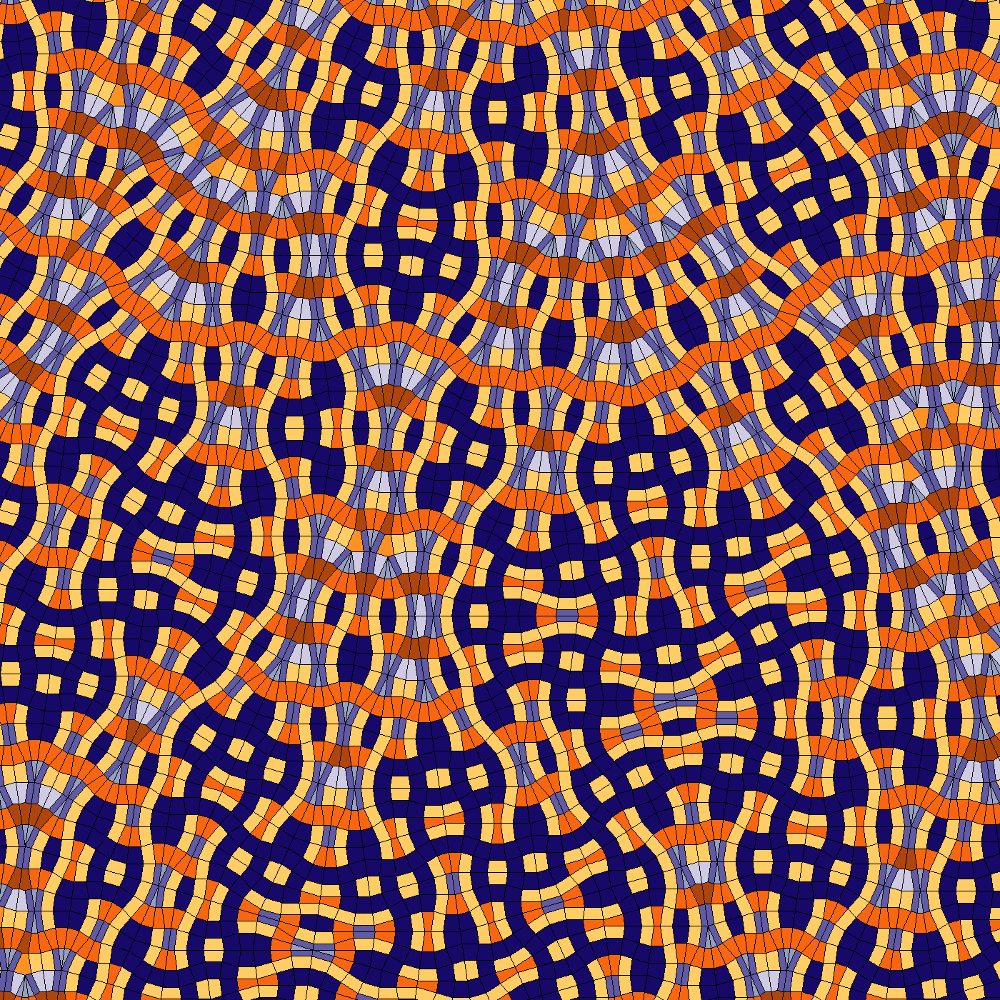## Cyclotomic Trapezoids 11-fold

### Info

In order to generalize Danzer’s 7-fold tiling to n-fold symmetry, where n>5 is odd, L. Danzer and D. Frettlöh introduced trapezoidal tiles, each one the union of two triangles with edge lengths of the form $\sin(k \frac{\pi}{n})$. It needs some further effort, including the introduction of three additional prototiles (two pentagons, one non-trapezoidal quadrangle), but one obtains an infinite series of substitution rules based on n-fold symmetry (n odd). Unfortunately, none of these tilings show perfect n-fold symmetry, as Danzer’s 7-fold does, thus loosing aesthetic appeal.

### Substitution Rule### Patch